Next: Is there any efficient Up: Convex Polyhedron Previous: Is there an efficient   Contents

## How can one remove all interior points of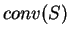from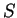for large cloudsof points in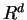?

The problem is formally known as the redundancy removal. Letbe a set of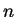points in. We say a pointis redundant (for) if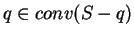. In short, redundant points are unnecessary to determine the convex hull.

In principle, one can apply the linear programming (LP) method given in 2.19 to remove all redundant points. This amounts to solvingLPs. While the time complexity of this pure LP method is polynomial and additional techniques given by [Cla94,OSS95] can reduce the size of LPs, this might end up in a very time consuming job for large(say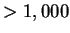).

There is a technique that might be useful to remove obviously redundant'' points quickly as a preprocessing. This works only in small dimensions (probably up to?). Such a method picks up a few nonredundant point set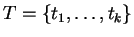from. Selecting nonredundant points can be done by picking points maximizing (or minimizing) any given linear function over. When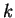is small relative to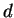, say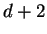or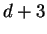, the computation of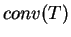is usually very easy with any standard convex hull algorithm. Thus we assume that an inequality system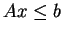such thatis given. It is easy to see that any point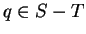satisfying the inequalities (i.e.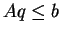) is redundant. One can repeat the same procedure with a different set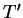of nonredundant points as long as it removes sufficient number'' of redundant points.Next: Is there any efficient Up: Convex Polyhedron Previous: Is there an efficient   Contents
Komei Fukuda 2004-08-26